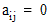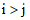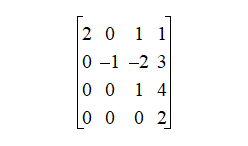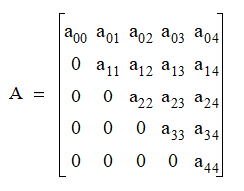# NMath User's Guide

17.2 Upper Triangular Matrices (.NET, C#, CSharp, VB, Visual Basic, F#)

An upper triangular matrix is a square matrix with all elements below the main diagonal equal to zero. That is,for. For example, this is a 4 x 4 upper triangular matrix:Like lower triangular matrices, upper triangular matrices often arise at an intermediate stage in solving systems of equations and inverting matrices.

NMath provides upper triangular matrix classes for four datatypes: single- and double-precision floating point numbers, and single- and double-precision complex numbers. The classnames are , , , and .

For efficiency, zero elements below the main diagonal are not stored. Instead, matrix values are stored in a vector column by column. For example, the following 5 x 5 upper triangular matrix:is stored in a data vector as:

```v = [ a00 a01 a11 a02 a12 a22 a03 a13 a23 a33 a04 a14 a24 a34 a44 ]
```

In general, the relationship between matrix and vector indices is:

```A[i,j] = v[i + j(j+1)/2]
```

Top

Top